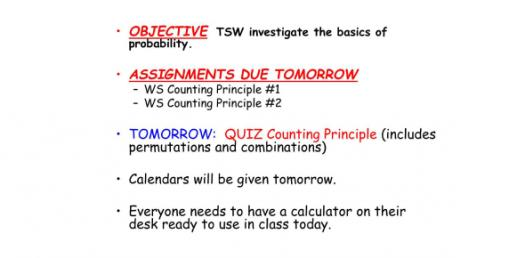# AP Statistics Quiz! Trivia Questions

5 Questions | Total Attempts: 666Settings.

• 1.
A set of test scores has the following 5-number summary:  Min  Q1  Median  Q3  Max  2  18  22.5  28.5  33 Which statement about outliers must be true?
• A.

There is exactly one outlier on the lower end.

• B.

There is at least one outlier on the lower end.

• C.

There is exactly one outlier on the higher end.

• D.

There is at least one outlier on the higher end.

• E.

There are no outliers.

• 2.
The table below shows the regression output for predicting the number of calories from the number of milligrams of sodium for items at a fast-food chain. What percent of the variation in calories is explained by the regression model using sodium as a predictor?
• A.

7.36%

• B.

54.2%

• C.

53.8%

• D.

73.4%

• E.

73.6%

• 3.
Marine biologists want to determine whether a small increase in acidity of sea water would adversely affect marine life.  They randomly assigned several Entacmaea quadricolor (a type of sea anemone), to one of eight aquariums with environments as similar as possible to the natural habitat.  Then they randomly selected four tanks, and gradually increased the acidity of the water.  They monitored the health of the anemones for the duration of the study.  Which is not true about this study?
• A.

An advantage of using only one variety of sea anemones is that there should be less variability in the response to each treatment.

• B.

A disadvantage of using only one variety of sea anemones is that the scope of inference is limited only to that type of anemone.

• C.

An advantage of using aquariums is that it is easier to maintain control to avoid confounding factors.

• D.

A disadvantage of using aquariums is that the anemones might respond differently in the ocean than they do in the aquariums.

• E.

If the anemones in the aquariums with increased acidity are less healthy than those without increased acidity, it cannot be determined that the increased acidity caused the response.

• 4.
A polling company wants to estimate, using a 95% confidence interval, the proportion of likely voters that would vote for a particular candidate in the upcoming election.  Of the following choices, which is the smallest sample size that will ensure a margin of sampling error less than or equal to 2 percentage points?
• A.

250

• B.

950

• C.

1,150

• D.

2,250

• E.

2,450

• 5.
A multiple choice test has 30 questions, and each question has five options.  A student has not studied and will guess on every question.  Which calculation shows how to find the probability of getting 15 or more questions correct?
• A.

A

• B.

B

• C.

C

• D.

D

• E.

E

Related TopicsBack to top